# Average mark

Calculate average mark in English, if a student got a couple of 3's, 20% less 2 than 3, and 50% more 1's than the 2's.

p =  1.9333

### Step-by-step explanation:

$p=\frac{3\cdot x+2\cdot 0.8x+1\cdot 1.5\cdot 0.8x}{x+0.8x+1.5\cdot 0.8x}=\frac{3\cdot 1+2\cdot 0.8+1\cdot 1.5\cdot 0.8}{1+0.8+1.5\cdot 0.8}=1.9333$Did you find an error or inaccuracy? Feel free to write us. Thank you!Tips to related online calculators
Looking for help with calculating arithmetic mean?
Looking for a statistical calculator?

#### You need to know the following knowledge to solve this word math problem:

We encourage you to watch this tutorial video on this math problem:

## Related math problems and questions:

• Crowns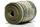1180 crowns are divided into three people: A got 20% less than B and C by 15% more than B. How many got A, B, and C?
• Final examThere are 5 learners in a class that has written a final exam Aleta scored 55% vera scored 36% and Sibusiso scored 88% if Thoko scored 71% and the class average was 63%. What was Davids score as a percentage?
• PeroxideHow many ml should we pour 30% of peroxide (H2O2) into 100ml H2O to give a 20% solution?
• Energy savingThey were released three different, independent inventions saving 20%, 24% and 15% energy. Some considered that while the use of these inventions, the total savings will be 20% + 24% + 15% = 59%. Is this true? How much percent of energy will save all thre
• RewardThree workers shared a common reward 13110 CZK: the first worker got 35% less than the second, and the third worker got 20% more than the second worker. How much got each worker?
• Dividing moneyThomas, Honza and Vasek are to divide 1220kč. Honza got 25% more than Thomas. Vasek 20% less than Thomas. How CZK each got?
• Salary in enterprise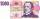The average salary in the company is 27 000 CZK, 30% of workers have the lowest average income of 19 thousand CZK. There were an increase in the salary in this group by 2%. How much % increased the average salary across the company?
• MegapizzaMegapizza will be divided among 100 people. First gets 1%, 2nd 2% of the remainder, 3rd 3% of the remainder, etc. Last 100th 100% of the remainder. Which person got the biggest portion?
• Geography testsOn three 150-point geography tests, you earned grades of 88%, 94%, and 90%. The final test is worth 250 points. What percent do you need on the final to earn 93% of the total points on all tests?
• Certificate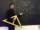There is 31 students in a class. From mathematics was'nt worse mark than 2. Average mark in mathematics was 2. How many students have mark 1 and how many mark 2?
• MeanA student's food the mean of 50 items as 38.6. When checking the work, he found that he had taken one item as 50 to read as 40 in these circumstances correctly. What should be the correct mean?
• Three workers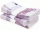The three workers received € 2,850 together for the work done. They divided them according to the time worked so that the first received 20% less than the second and the third € 50 more than the second. How much EUR did each worker receive?
• School year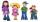At the end of the school year has awarded 20% of the 250 children who attend school. Award got 18% boys and 23% of girls. Find how many boys and how many girls attend school.
• Four pupilsFour pupils divided $1485 so that the second received 50% less than the first, the third 1/2 less than a fourth, and fourth$ 154 less than the first. How much money had each of them?
• Percents from percentHow much is 13% of 20% of 500 greater than 8% of 14% of 200?
• 3y inflation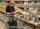Price of the roll rise in the first year by 9%, the second year fell by 5% and in the third year increased by 3%. Calculate the average annual increase in price of the roll.
• The notebook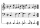After rising by 40%, the notebook cost 10.50 euros. How much did this notebook cost if it increased in price by only 20% instead of 40%?